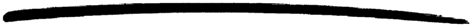## 7 Macros

### 7.1 Defining and Using Macros

A macro is defined the following way:

```-define(Const, Replacement).
-define(Func(Var1,...,VarN), Replacement).

```

A macro definition can be placed anyhere among the attributes and function declarations of a module, but the definition must come before any usage of the macro.

If a macro is used in several modules, it is recommended that the macro definition is placed in an include file.

A macro is used the following way:

```?Const
?Func(Arg1,...,ArgN)

```

Macros are expanded during compilation. A simple macro `?Const` will be replaced with `Replacement`. Example:

```-define(TIMEOUT, 200).
...
call(Request) ->
server:call(refserver, Request, ?TIMEOUT).

```

This will be expanded to:

```call(Request) ->
server:call(refserver, Request, 200).

```

A macro `?Func(Arg1,...,ArgN)` will be replaced with `Replacement`, where all occurences of a variable `Var` from the macro definition are replaced with the corresponding argument `Arg`. Example:

```-define(MACRO1(X, Y), {a, X, b, Y}).
...
bar(X) ->
?MACRO1(a, b),
?MACRO1(X, 123)

```

This will be expanded to:

```bar(X) ->
{a,a,b,b},
{a,X,b,123}.

```

It is good programming practice, but not mandatory, to ensure that a macro definition is a valid Erlang syntactic form.

To view the result of macro expansion, a module can be compiled with the `'P'` option. `compile:file(File, ['P'])`. This produces a listing of the parsed code after preprocessing and parse transforms, in the file `File.P`.

### 7.2 Predefined Macros

The following macros are predefined:

`?MODULE`
The name of the current module.
`?MODULE_STRING`.
The name of the current module, as a string.
`?FILE`.
The file name of the current module.
`?LINE`.
The current line number.
`?MACHINE`.
The machine name, `'BEAM'`.

### 7.3 Flow Control in Macros

The following macro directives are supplied:

`-undef(Macro).`
Causes the macro to behave as if it had never been defined.
`-ifdef(Macro).`
Evaluate the following lines only if `Macro` is defined.
`-ifndef(Macro).`
Evaluate the following lines only if `Macro` is not defined.
`-else.`
Only allowed after an `ifdef` or `ifndef` directive. If that condition was false, the lines following `else` are evaluated instead.
`-endif.`
Specifies the end of an `ifdef` or `ifndef` directive.

Example:

```-module(m).
...

-ifdef(debug).
-define(LOG(X), io:format("{~p,~p}: ~p~n", [?MODULE,?LINE,X])).
-else.
-define(LOG(X), true).
-endif.

...

```

When trace output is desired, `debug` should be defined when the module `m` is compiled:

```% erlc -Ddebug m.erl

or

1> c(m, {d, debug}).
{ok,m}

```

`?LOG(Arg)` will then expand to a call to `io:format/2` and provide the user with some simple trace output.

### 7.4 Stringifying Macro Arguments

The construction `??Arg`, where `Arg` is a macro argument, will be expanded to a string containing the tokens of the argument. This is similar to the `#arg` stringifying construction in C.

The feature was added in Erlang 5.0/OTP R7.

Example:

```-define(TESTCALL(Call), io:format("Call ~s: ~w~n", [??Call, Call])).

?TESTCALL(myfunction(1,2)),
?TESTCALL(you:function(2,1)).

```

results in

```io:format("Call ~s: ~w~n",["myfunction ( 1 , 2 )",m:myfunction(1,2)]),
io:format("Call ~s: ~w~n",["you : function ( 2 , 1 )",you:function(2,1)]).

```

That is, a trace output with both the function called and the resulting value.